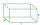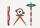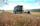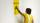# Drawer

The rectangular face of a drawer has a perimeter of 108 cm and a width of 18cm. Find the length and the area of the rectangular face of the drawer.

Result

b =  36 cm
A =  648 cm2

#### Solution:Leave us a comment of example and its solution (i.e. if it is still somewhat unclear...):Be the first to comment!## Next similar examples:

1. A rectangularA rectangular garden 40 m long and 30 m wide is to be fenced with fence posts at each corner. All the other posts will be 5 meters a part. How many posts will be needed to fence in the garden.
2. LandLand has a rectangular shape, its surface area is 1.45 hectares. Its width is 250 m. Determine the length of the land.
3. Metal railsDad needs to improve the edges of the wooden boxes - to be reinforced with metal rails. How many cms of rails will he need if the box has the shape of a prism with the length of the edges 70cm 70cm and 120cm?
4. Circle r,DCalculate the diameter and radius of the circle if it has length 52.45 cm.
5. Circle simpleCircumference of a circle is 6.28. What is the area of the circle?
6. CircleWhat is the radius of the circle whose perimeter is 6 cm?
7. ParallelogramCalculate the missing side of a parallelogram, if you know the perimeter o and one side: a) o = 7.2 cm; b = 1.8 cm b) o = 5.4 cm; a = 1.9 cm
8. Bicycle wheelAfter driving 157 m bicycle wheel rotates 100 times. What is the radius of the wheel in cm?
9. Field on planPlan has a scale of 1: 2500.Determine dimensions in centimeters which will have on plan field with a length of 310 meters and a width of 182.5 meters.
10. Bathroom 2A bathroom is 2.4 meters long and 1.8 meters wide. How many square tiles 1 dm on each side are to be used to cover it?
11. FarmlandFarmers seeded maize to 45 ha, which is 15% of their total farmland. How many hectares of fields they have?
12. Wall paintingThe wall is 5 meters wide and 2 meters high. Window in the wall has dimensions 2x1,8 meters. How many litera of color is needed to paint two-layer this walls, if the 1 m2 needs 1.1 liters of paint?
13. GardenThe garden around the new majer is divided as follows: 35% vegetable, 30% fruit orchards, 10% flowers and the remaining 120 m2 are lawns. What is the total area of the garden?
14. RainfallHow many liters of water did fell in a 32m long and 8m wide garden, if 8mm of rain fell?
15. Simple equationSolve for x: 3(x + 2) = x - 18
16. Seeds 2How many seeds germinated from 1000 pcs, when 23% no emergence?
17. BicycleThe bicycle pedal gear has 36 teeth, the rear gear wheel has 10 teeth. How many times turns rear wheel, when pedal wheel turns 120x?